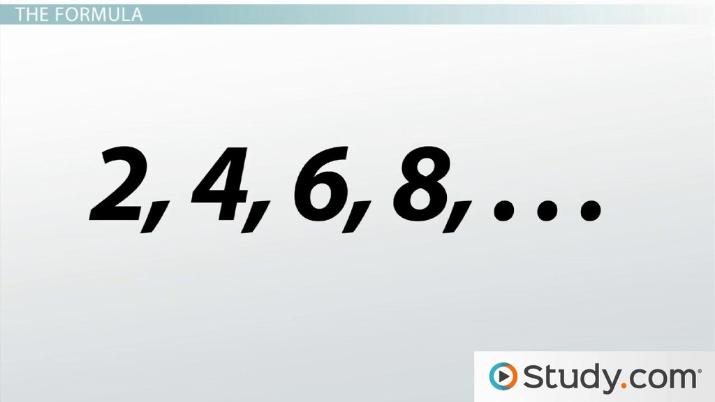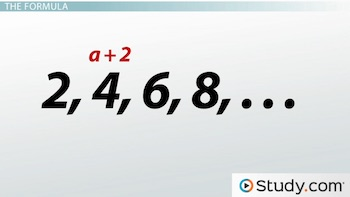Arithmetic Sequences: Definition & Finding the Common Difference

Lesson Transcript
Instructor: Yuanxin (Amy) Yang Alcocer

Amy has a master's degree in secondary education and has been teaching math for over 9 years. Amy has worked with students at all levels from those with special needs to those that are gifted.

Arithmetic sequences are a string of numbers where each number is the previous number plus a constant, called the common difference. Learn more about it's definition and finding the common difference. Updated: 10/06/2021

What Is an Arithmetic Sequence?

What is an arithmetic sequence? It is a string of numbers where each number is the previous number plus a constant, called the common difference. Think of an arithmetic sequence as if you are hosting a candy party where each person that comes is required to bring two candy bars. You are already there with two candy bars. The next person that comes brings two more candy bars. Now you have four candy bars. The next person that comes brings two more candy bars. Now your total is six candy bars. You can see a pattern forming here, and you can probably continue this pattern on your own.An error occurred trying to load this video.

Try refreshing the page, or contact customer support.

Coming up next: How and Why to Use the General Term of an Arithmetic Sequence

You're on a roll. Keep up the good work!

Replay
Your next lesson will play in 10 seconds
• 0:01 What Is an Arithmetic…
• 0:35 Sequence Examples
• 1:22 Finding the Common Difference
• 2:38 The Formula
• 5:08 Lesson Summary
Save Save

Want to watch this again later?

Timeline
Autoplay
Autoplay
Speed Speed

Sequence Examples

The candy bar example gives you the sequence of 2, 4, 6. We can end there if only two guests came to the party. But we could also continue the pattern indefinitely if we wanted to. We can continue it by adding 2 to 6 to get 8, then adding 2 to 8 to get 10. We would get 2, 4, 6, 8, 10, . . . etc.

Other examples of arithmetic sequences include:

1, 2, 3, 4, . . . etc.

2, 5, 8, 11, . . . etc.

3, 5, 7, 9, . . . and so forth

Notice how all of these sequences have numbers where each number is the previous number plus a constant, a common difference.

Finding the Common Difference

We can calculate the common difference for each of our sequences by taking any two numbers that are next to each other and then subtracting the first from the second. We can repeat with another pair of numbers to make sure that the difference is the same. So for our first sequence of 1, 2, 3, 4, . . ., we can subtract the 1 from the 2 to get 2 - 1 = 1. We can also subtract the 2 from the 3 to get 3 - 2 = 1. Look at that! The common difference is the same - just what we would expect! If we repeated this process with the 3 and the 4, we would see that it also has a difference of 1, so this arithmetic sequence has a common difference of 1.

The second sequence, 2, 5, 8, 11, . . ., has a common difference of 3 because when we subtract the 2 from the 5, we get 3. We also get 3 when we subtract the 5 from the 8 and the 8 from the 11.

Can you find the common difference for the third sequence, 3, 5, 7, 9, etc . . .? If we subtract the 3 from the 5 and the 5 from the 7, we get 2. 7 subtracted from 9 is also 2. So the common difference for this sequence is 2.

The Formula

Because we have a common difference between all the numbers in our arithmetic sequence, we can use this information to create a formula that allows us to find any number in our sequence, whether it is the 10th number or the 50th number. If you think about it, each number in an arithmetic sequence is actually the first number plus the common difference multiplied by how many times we needed to add it. See, to get to the second term, we added the common difference once to the first term:To unlock this lesson you must be a Study.com Member.

Register to view this lesson

Are you a student or a teacher?